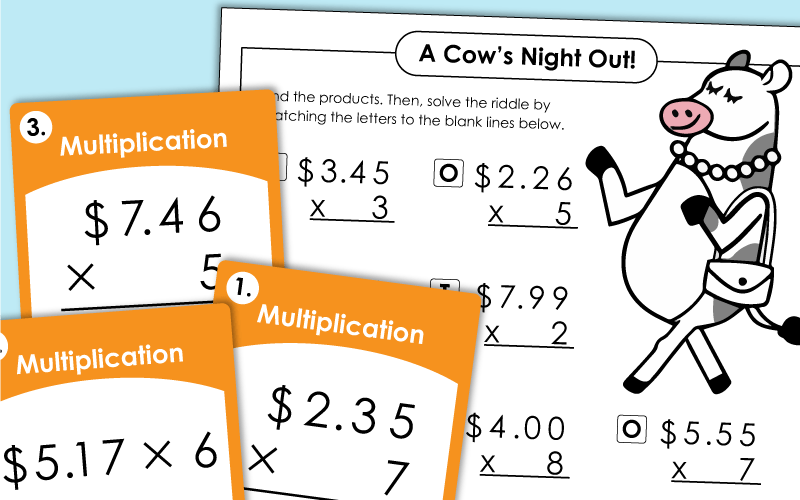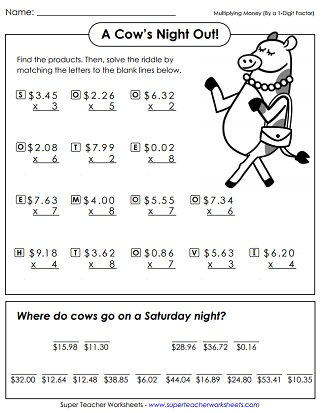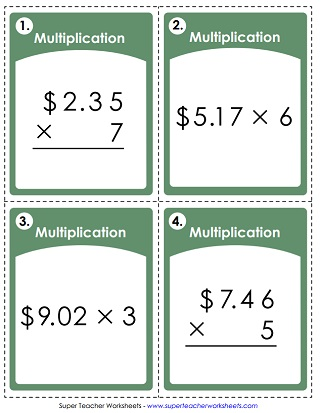# Multiplying Money Worksheets

These printables allow students to review and practice finding the products of a money amount and a whole number. (example: \$3.65 x 7)## Money Times 1-DigitWorksheets

Find the cost of the bakery items shown.  Multiply money amounts by 1-digit numbers. (example: \$1.06 x 4)
Where do cows go on Saturday evenings? To find the answer to this funny riddle, solve the multiplication problems. Each problem requires students to multiply a money amount by a single-digit number.
At the top of this page, kids will see a collection of polygons with numbers or money amounts in them. Students multiply pairs of similar shapes. For example: Find the product of the numbers in the hexagons. \$3.12 x 5
Calculate how much it would cost to buy items at a fruit stand.  Requires multiplication of money by 1-digit numbers. (example: \$0.37 x 3)
Calculate how much it would cost to purchase several items from a fruit stand.  Multiple step problems require multiplication and addition.
Multiplication problems with money amounts; Winter snowmen pictures
This worksheet has several types of money multiplication problems, including a multi-step word problem and an input/output table.
Graph Paper Math Drills; Dollars and cents times 1-digit; (example: \$4.99 x 3)
Use the toy shop picture to answer the multiplication problems. Dollars and cents times 1-digit (example: \$7.40 x 4)

## Multi-Digit MultiplicationWorksheet Generator

Multiplication Worksheet Generator

Create your own multiplication worksheets! You choose the number of digits in the factors. Also tell if you would like to include money or word problems.

This file has a set of 30 task cards. Half of the problems are vertical and the other half if horizontal. Use these cards for peer practice sessions, learning centers, or on your classroom document reader.
More Multi-Digit Multiplication

We invite you to browse our massive (and still growing!) collection of multi-digit multiplication worksheets.

## Pictures of Our WorksheetsMy Account
Site Information